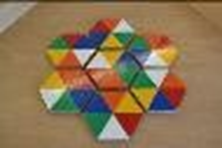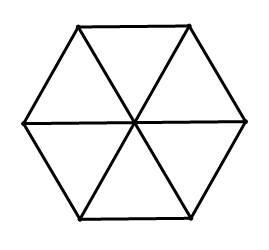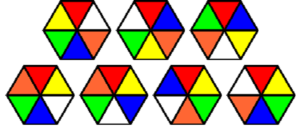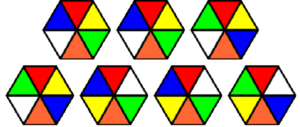# HoneycombHow many colours can you match around the centre hexagon? Can you match all six free hexagons around the centre hexagon? How many possible ways are there to arrange the hexagons?
Maths

Consider how many ways we can arrange the hexagons around centre. We have 6 choices for the first hexagon, then 5 choices left for the second hexagon, then 4 choices, then 3, then 2 and finally 1. To calculate how many ways to arrange the seven hexagons we multiply our choices together, so this number is 6×5×4×3×2×1 = 720.
Each hexagon can be rotated six ways, so that’s another 66 choices, so altogether there are 720×66 = 33,592,320 possibilities. That’s a lot. If we exclude rotations of the whole puzzle, there are still 5,598,720 possibilities.
Fortunately, it’s not quite as bad as it seems. Many of our choices are forced, because we are trying to match colours, so most possibilities can be quickly rejected. There are actually fewer than 10 possibilities to check. If all hexagons are free the number of possibilities is still fewer than 50. There is only one solution.

History

This puzzle is called the Circus Seven, as the colourful hexagons look a bit like circus tents. At first this puzzle may seem very difficult, but most possibilities can be quickly rejected, leaving only a few possibilities to check.

People
Applications

This puzzle is similar to a Rubik Cube because there are a lot of possibilities, but only one correct answer. Both puzzles are applications of combinatorics.
Combinatorics is the study of counting arrangements, constructing solutions to problems and studying their structure, and often uses diagrams, such as networks, to solves problems. Combinatorics is used a lot in computer science to create networks of computers and to order information.

Maths at Home

Colour in this hexagon and make your own honeycomb puzzle at home.Here are the colours you need:The honeycomb puzzle is sometimes called Circus Seven. Here are some instructions to make an origami version of the puzzle:

Here are some variations of the puzzle. Try them yourself. Do you think they are harder, easier, or the same as before?

This variation is called Drive Me Nuts:And this variation is called Circus Puzzler: